This resource function is obsolete. Use the paclet Wolfram/QuantumFramework instead.

Function Repository Resource:

# QuantumEntanglementMonotone

Compute the degree of entanglement between a pair of subsystems in a discrete quantum state

Contributed by: Jonathan Gorard and Taufiq Murtadho
 ResourceFunction["QuantumEntanglementMonotone"][QuantumDiscreteState[…],bipart] computes the generalized concurrence monotone for the subsystems in the bipartition bipart of the specified QuantumDiscreteState. ResourceFunction["QuantumEntanglementMonotone"][QuantumDiscreteState[…],bipart,mono] computes the named entanglement monotone mono for the subsystems in the bipartition bipart of the specified QuantumDiscreteState. ResourceFunction["QuantumEntanglementMonotone"][QuantumDiscreteState[…],bipart,{mono,α}] computes the named entanglement monotone mono with index α (where appropriate) for the subsystems in the bipartition bipart of the specified QuantumDiscreteState.

## Details

Whenever the entanglement monotone mono is not specified, "Concurrence" is assumed by default.
Whenever the bipartition bipart is not specified, the first and second subsystems (as specified by the bipartition {{1},{2}}) are assumed by default.
In ResourceFunction["QuantumEntanglementMonotone"][QuantumDiscreteState[],], the specified QuantumDiscreteState should contain at least two subsystems (qudits).
The bipartition bipart should be a nested list of the general form {{i1,i2,},{j1,j2,}}, with positive integers i1, i2,, j1, j2, specifying subsystems of the given QuantumDiscreteState. For instance, the bipartition {{1,2},{3}} partitions the QuantumDiscreteState into subsystems {1,2} and {3}.
In ResourceFunction["QuantumEntanglementMonotone"][QuantumDiscreteState[],bipart,mono], the following entanglement monotones mono are supported:
 "Concurrence" generalized concurrence monotone for pure states and two-subsystem mixed states "Negativity" negativity monotone for pure and mixed states (i.e. the Peres–Horodecki separability criterion) "LogNegativity" logarithmic negativity monotone for pure and mixed states (i.e. the Peres–Horodecki separability criterion) "EntanglementEntropy" entanglement entropy monotone for pure states (i.e. the von Neumann entropy of the reduced density matrices) "RenyiEntanglementEntropy" Rényi entanglement entropy monotone with Rényi index α=1/2 for pure states (i.e. the Rényi entropy of the reduced density matrices) {"RenyiEntanglementEntropy",α} Rényi entanglement entropy monotone with specified Rényi index α for pure states (i.e. the Rényi entropy of the reduced density matrices)
Whenever the entanglement monotone mono is not defined for the specified QuantumDiscreteState, ResourceFunction["QuantumEntanglementMonotone"] will return Indeterminate.

## Examples

### Basic Examples (5)

Compute the generalized concurrence monotone for the first and second subsystems of a two-qubit pure discrete quantum state:

 In:=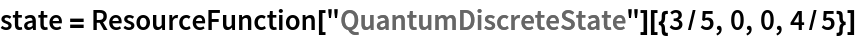Out=In:=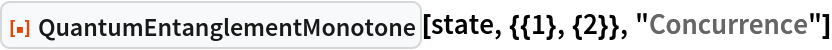Out=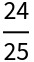Compute the negativity and logarithmic negativity monotones instead:

 In:=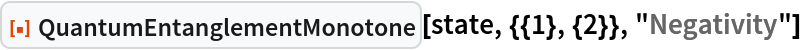Out=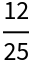In:=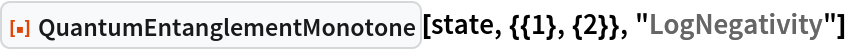Out=Compute the entanglement entropy and Rényi entanglement entropy monotones instead:

 In:=Out=In:=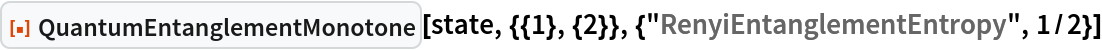Out=In:=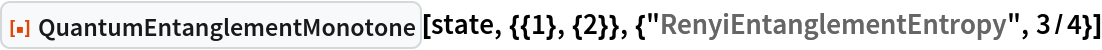Out=Show that the three-qubit GHZ state is biseparable (i.e. any pair of qubits is separable):

 In:=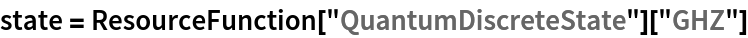Out=In:=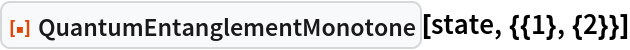Out=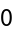In:=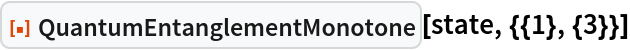Out=In:=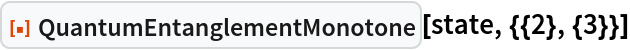Out=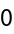However, the GHZ state is not triseparable (since every triple of qubits is maximally entangled):

 In:=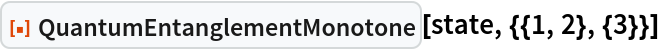Out=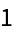Show that the three-qubit W state is not biseparable (and hence its entanglement is more robust than the GHZ state's):

 In:=Out=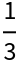Show that a QuantumDiscreteState consisting of two three-dimensional qudits is not separable:

 In:=Out=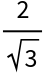Compute negativity and logarithmic negativity entanglement monotones for subsystems {2,3} and {5} in a random 5-qubit pure state:

 In:=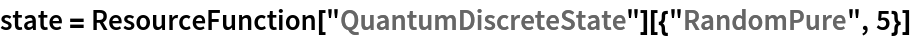Out=In:=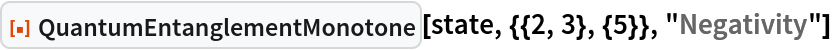Out=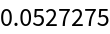In:=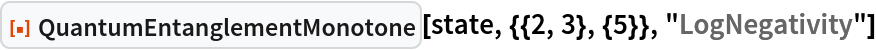Out=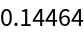### Scope (2)

The concurrence monotone is defined for mixed states, but only for pairs of subsystems:

 In:=Out=In:=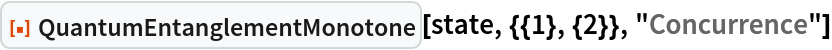Out=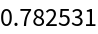For any triple of subsystems, the concurrence monotone is undefined (returns Indeterminate):

 In:=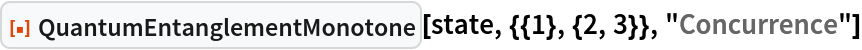Out=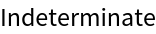On the other hand, the negativity and logarithmic negativity monotones are always defined for mixed states, for any collection of subsystems (of any size):

 In:=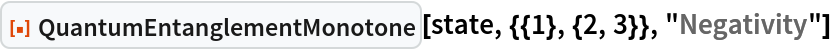Out=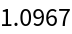In:=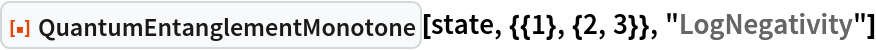Out=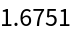The entanglement entropy and Rényi entanglement entropy monotones are never defined for mixed states:

 In:=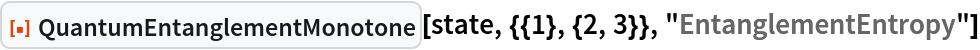Out=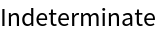In:=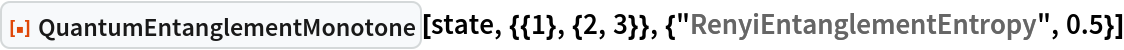Out=The Rényi entanglement entropy approaches the standard (von Neumann) entanglement entropy in the limit as α approaches 1:

 In:=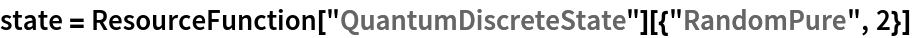Out=In:=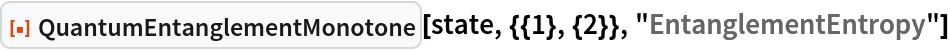Out=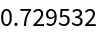In:=Out=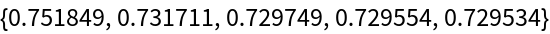Jonathan Gorard

## Version History

• 1.0.0 – 26 April 2021